0+0+0+0+0+0+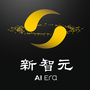0+0+0+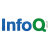0+0+0+0+0+0+0+0+0+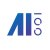【从YARN迁移到k8s，滴滴机器学习平台二次开发是这样做的】从YARN迁移到k8s，滴滴机器学习平台二次开发是这样做的 在2019 #AI开发者大会# 上，滴滴出行资深软件工程师唐博在机器学习技术分论坛上分享了kubernetes调度系统在滴滴在机器学习平台中的落地与二次开发。0+0+0+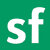0+0+0+0+0+0+0+0+0+0+0+0+0+0+0+0+0+0+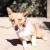0+0+0+0+0+0+
Top